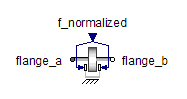Electrical Power Sensor - MapleSim Help

Electrical Power Sensor

Sense three-phase powerDescription The Electrical Power Sensor component converts three-phase instantaneous voltages (from ${\mathrm{plug}}_{p}$  to ${\mathrm{plug}}_{\mathrm{nv}}$) and currents (from ${\mathrm{plug}}_{p}$ to ${\mathrm{plug}}_{\mathrm{ni}}$) to corresponding space phasors. These space phasors are used to calculate instantaneous power or stationary state active power (P) and the stationary state reactive power (Q).Connections

 Name Description Modelica ID ${\mathrm{plug}}_{p}$ Positive electrical 3-phase plug plug_p ${\mathrm{plug}}_{\mathrm{ni}}$ Negative electrical 3-phase plug for current plug_ni ${\mathrm{plug}}_{\mathrm{nv}}$ Negative electrical 3-phase plug for voltage plug_nv $P$ Real output; active power in $W$ P $Q$ Real output; reactive power in $\mathrm{VA}$ QModelica Standard Library The component described in this topic is from the Modelica Standard Library. To view the original documentation, which includes author and copyright information, click here.